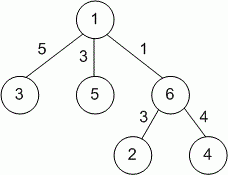Competitions

# Tree

A weighted tree is a tree where each edge is labeled with a number representing the edge's length. All lengths are positive. For each node, you have to find the maximum possible distance to any other node in the tree.

#### Input

Contains the description of the tree. The first line contains the number of vertices n (2n50000) in a tree. Each of the following (n - 1) lines contains the description of the tree's edges. Each edge is described by three positive integers. The first two integers are the labels of the nodes connected by this edge, ranging from 1 to n, the third number - the length of the edge. The total length of all edges does not exceed 231 - 1. It is guaranteed that the description of the tree is correct.

#### Output

Print exactly n lines: the k-th line contains the distance from node k (k = 1..n) to the most distant node.Time limit 1 second
Memory limit 128 MiB
Input example #1
6
1 5 3
2 6 3
6 1 1
1 3 5
4 6 4

Output example #1
5
9
10
10
8
6

Source ACM ICPC SEERC-2012, 13.10.2012 Bucharest, Vinnica